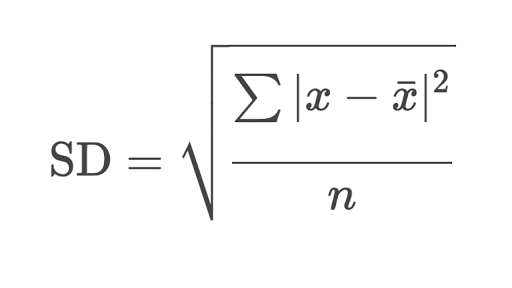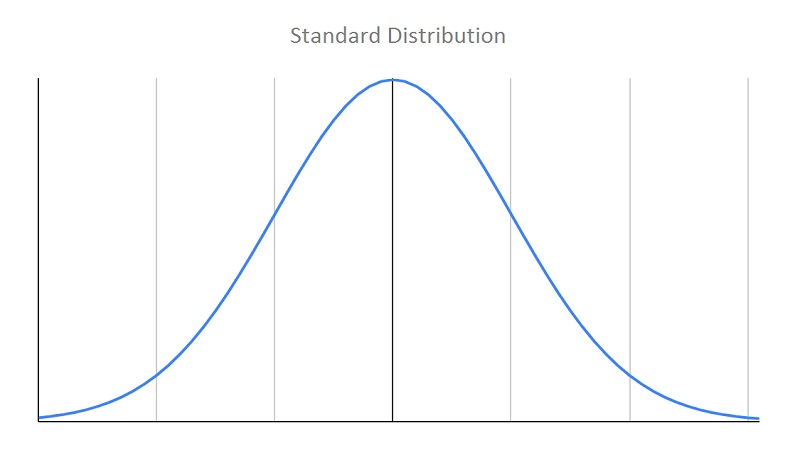﻿ Standard Deviation Calculator | Online Average Deviation Tool

# Standard Deviation Calculator

The standard deviation is a measure of how precise the average is, that is, how well the individual numbers agree with each other. Use our online standard deviation calculator, a free standard deviation tool to find deviation of the given set of the numbers.

## Standard Deviation Formulax is the set of the numbers

x̄ or mean is the average of the set of the numbers

n is the size of the set

SD or σ is the standard deviation

This formula indicates that the standard deviation is the square root of an average. This average is the average of the squared differences between each value and the mean. The differences are squared to remove the sign so that negative values will not cancel out positive values. After summing up these squared differences and dividing by N, the square root is taken to give the result in the original scale. That is, the standard deviation can be thought of as the average difference between the data values and their mean (the terms mean and average are used interchangeably).

### How Standard Deviation is Calculated

It is difficult to understand the standard deviation solely from the standard deviation formula. There are two general interpretations that can be useful in understanding the standard deviation.

1. The standard deviation may be thought of as the average difference between an observation and the mean, ignoring the sign.

2. The standard deviation may be thought of as the average difference between any two data values, ignoring the sign.### Application of Standard Deviation

• The standard deviation enables us to determine with a great deal of accuracy, where the values of a frequency distribution are located in relation to the mean.
• Summarizes the deviation of a large distribution from mean in one figure used as a unit of variation.
• Indicates weather the variation is real or due to special reason
• Helps in comparing two samples
• Helps in finding the suitable sample size for valid conclusion### Spreading Knowledge Across the World

USA - United States of America  Canada  United Kingdom  Australia  New Zealand  South America  Brazil  Portugal  Netherland  South Africa  Ethiopia  Zambia  Singapore  Malaysia  India  China  UAE - Saudi Arabia  Qatar  Oman  Kuwait  Bahrain  Dubai  Israil  England  Scotland  Norway  Ireland  Denmark  France  Spain  Poland  and  many more....